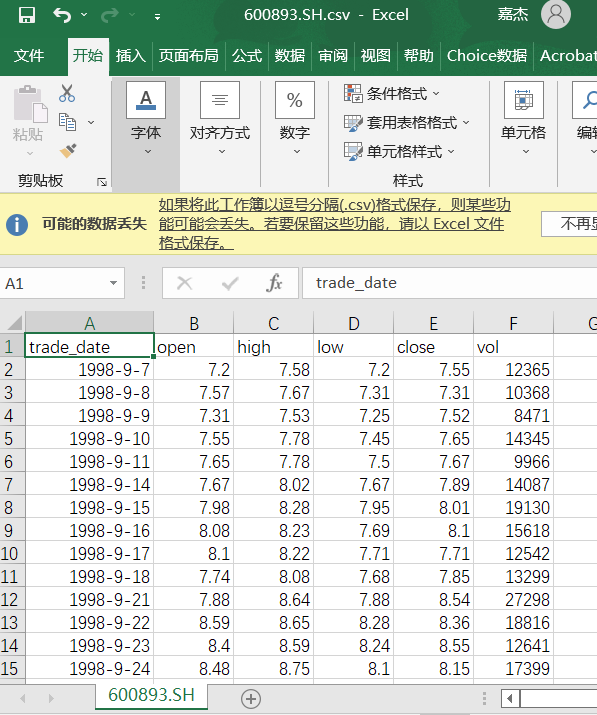# 利用Tushare获取股票数据（全面详细，照着敲就可以）

tushare是一个免费,开源的python财经数据接口包.主要实现对股票等金融数据从数据采集、清洗加工到数据存储的过程，能够为金融分析人员提供快速、整洁、和多样的便于分析的数据，为他们在数据获取方面极大地减轻工作量，使他们更加专注于策略和模型的研究与实现上。考虑到Pythonpandas包在金融量化分析中体现出的优势，TuShare返回的绝大部分的数据格式都是pandasDataFrame类型，非常便于用pandas/NumPy/Matplotlib进行数据分析和可视化。当然，如果您习惯了用Excel或者关系型数据库做分析，您也可以通过TuShare的数据存储功能，将数据全部保存到本地后进行分析。

Tushare拥有丰富的数据内容，如股票、基金、期货、数字货币等行情数据，公司财务、基金经理等基本面数据。同时，SDK开发包支持语言，同时提供HTTP Restful接口，最大程度方便不同人群的使用。并且提供多种数据储存方式，如Oracle、MySQL，MongoDB、HDF5、CSV等，为数据获取提供了性能保证。

Tushare官网：Tushare大数据社区

pip install tushare

``````import tushare

print(tushare.__version__)``````

三.使用Tushare爬取股票数据

1.导入各种库（pandas、tushare、matplotlib库等）

``````import pandas as pd
import matplotlib.pyplot as plt
plt.rcParams['font.sans-serif'] = ['SimHei']
import tushare as ts
import os
import tushare as ts
ts.set_token('密匙')
pro = ts.pro_api()``````

2.设置导入数据格式、日期等，股票数据为前复权

``````def get_data(code,start,end):
df=pro.daily(ts_code=code,autype='qfq',start_date=start,end_date=end)
print(df)
#设置把日期作为索引
#df['ma'] = 0.0  # Backtrader需要用到
#df['openinterest'] = 0.0  # Backtrader需要用到
#定义两个新的列ma和openinterest
df = df[['open', 'high', 'low', 'close', 'vol']]
#重新设置df取值，并返回df
return df``````

3.下载股票数据，且用csv保存，保存至指定位置

``````def acquire_code():   #只下载一只股票数据，且只用CSV保存   未来可以有自己的数据库
inp_code =input("请输入股票代码:\n")
inp_start = input("请输入开始时间:'\n'")
inp_end = input("请输入结束时间:'\n'")
df = get_data(inp_code,inp_start,inp_end)
print(df.info())
#输出统计各列的数据量
print("—"*30)
#分割线
print(df.describe())
#输出常用统计参数
df.sort_index(inplace=True)
#把股票数据按照时间正序排列
path = os.path.join(os.path.join(os.getcwd(),
"文件夹名"), inp_code + ".csv")
#os.path地址拼接，''数据地址''为文件保存路径
# path = os.path.join(os.path.join(os.getcwd(),"数据地址"),inp_code+"_30M.csv")
df.to_csv(path)``````

4.运行函数，爬取股票数据

``acquire_code()``

5.输入股票名，数据起始、中止日期

``````请输入股票代码：

6.到指定文件夹位置查看爬取数据# 总结

Tushare是一个性能优异的大数据社区，同时高校学生和老师以及机构研究者均可获得免费积分进行数据的获取。